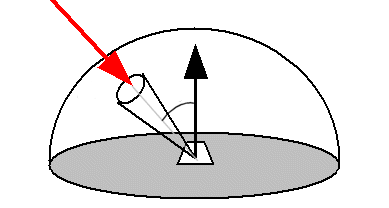A# DarlingIrradiance. Image credit: MIT.

Irradiance, also known as radiant flux density, is the total energy of radiation falling on a unit area of a surface from all directions above the surface per second. Since energy per unit time is power, we can also say that irradiance is the power incident on a unit area of the surface. Irradiance applies to both electromagnetic radiation and radioactivity. It is measured in watts per square meter (W/m2).

If P is the power and A the area of the surface, then I, the irradiance, is given by:

I = P/A.

As the the distance from a point source of radiation increases, the irradiance decreases. The relationship between irradiance and distance, d, follows an inverse square law:

I = k/d 2,

where k is a constant. It follows that, for two points at distances d1 and d2 from a point source of radiation:

I1d12 = I2d22.

## Irradiance in solar energy systems

Irradiance is a quantity often used when considering the design and operation of solar energy systems, in which case it is taken to mean the direct, diffuse, and reflected solar radiation that strikes each uit of a surface every second. Because solar irradiance is a measure of instantaneous power density, it varies throughout the day. Note that irradiance is not the same thing as insolation although they are measure in the same units. Solar insolation is the total amount of solar energy received at a location in a specified time (units usually kWh/(m2 day)). The units are effectively the same because insolation has time in both the numerator and denominator so it cancels out. Insolation is often used for simple photovoltaic system design and irradiance in more complex systems.

## Illuminance

Illuminance, also known as luminous flux density, is the total amount of light falling on a unit area of a surface from all directions above the surface in a given time. It is equivalent to irradiance weighted with the response curve of the human eye. Illuminance is measured in lux (lx), or lumens per square meter (lm/m2).